Instead of generating paths that maximize clearance, suppose that the goal is to find shortest paths. This leads to the shortest-path roadmap, which is also called the reduced visibility graph in . The idea was first introduced in  and may perhaps be the first example of a motion planning algorithm. The shortest-path roadmap is in direct conflict with maximum clearance because shortest paths tend to graze the corners of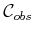. In fact, the problem is ill posed becauseis an open set. For any path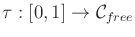, it is always possible to find a shorter one. For this reason, we must consider the problem of determining shortest paths in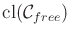, the closure of. This means that the robot is allowed to touch'' or graze'' the obstacles, but it is not allowed to penetrate them. To actually use the computed paths as solutions to a motion planning problem, they need to be slightly adjusted so that they come very close tobut do not make contact. This slightly increases the path length, but the additional cost can be made arbitrarily small as the path gets arbitrarily close to.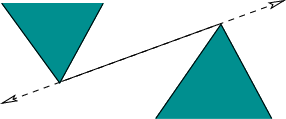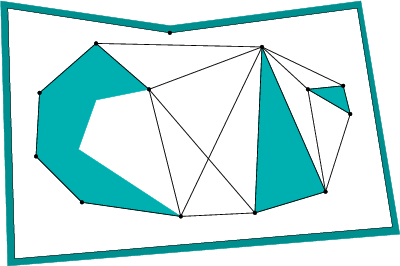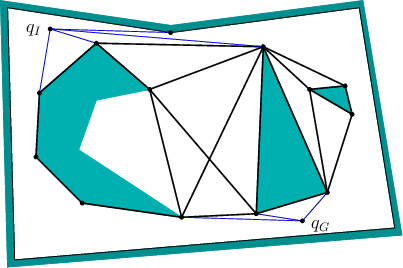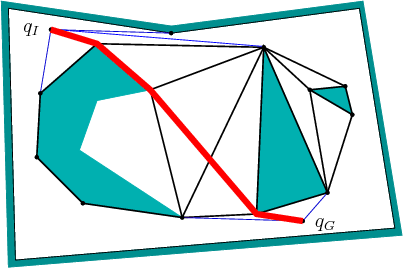The shortest-path roadmap,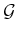, is constructed as follows. Let a reflex vertex be a polygon vertex for which the interior angle (in) is greater than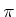. All vertices of a convex polygon (assuming that no three consecutive vertices are collinear) are reflex vertices. The vertices ofare the reflex vertices. Edges ofare formed from two different sources:

1. [] Consecutive reflex vertices: If two reflex vertices are the endpoints of an edge of, then an edge between them is made in.
2. [] Bitangent edges: If a bitangent line can be drawn through a pair of reflex vertices, then a corresponding edge is made in. A bitangent line, depicted in Figure 6.10, is a line that is incident to two reflex vertices and does not poke into the interior ofat any of these vertices. Furthermore, these vertices must be mutually visible from each other.
An example of the resulting roadmap is shown in Figure 6.11. Note that the roadmap may have isolated vertices, such as the one at the top of the figure. To solve a query,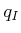and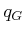are connected to all roadmap vertices that are visible; this is shown in Figure 6.12. This makes an extended roadmap that is searched for a solution. If Dijkstra's algorithm is used, and if each edge is given a cost that corresponds to its path length, then the resulting solution path is the shortest path betweenand. The shortest path for the example in Figure 6.12 is shown in Figure 6.13.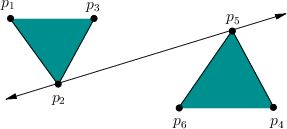If the bitangent tests are performed naively, then the resulting algorithm requires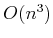time, in whichis the number of vertices of. There are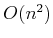pairs of reflex vertices that need to be checked, and each check requires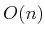time to make certain that no other edges prevent their mutual visibility. The plane-sweep principle from Section 6.2.2 can be adapted to obtain a better algorithm, which takes only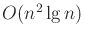time. The idea is to perform a radial sweep from each reflex vertex,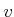. A ray is started at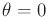, and events occur when the ray touches vertices. A set of bitangents throughcan be computed in this way in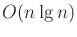time. Since there arereflex vertices, the total running time is. See Chapter 15 of  for more details. There exists an algorithm that can compute the shortest-path roadmap in time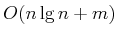, in which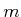is the total number of edges in the roadmap . If the obstacle region is described by a simple polygon, the time complexity can be reduced to; see  for many shortest-path variations and references.

To improve numerical robustness, the shortest-path roadmap can be implemented without the use of trigonometric functions. For a sequence of three points,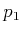,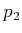,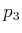, define the left-turn predicate,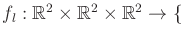TRUE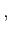FALSE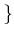, as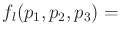TRUE if and only ifis to the left of the ray that starts atand pierces. A pointis a reflex vertex if and only ifTRUE, in whichandare the points before and after, respectively, along the boundary of. The bitangent test can be performed by assigning points as shown in Figure 6.14. Assume that no three points are collinear and the segment that connectsand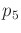is not in collision. The pair,,, of vertices should receive a bitangent edge if the following sentence is FALSE: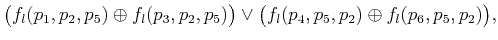(6.1)

in which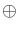denotes logical exclusive or.'' The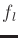predicate can be implemented without trigonometric functions by defining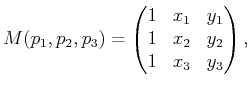(6.2)

in which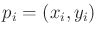. If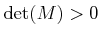, thenTRUE; otherwise,FALSE.

Steven M LaValle 2020-08-14